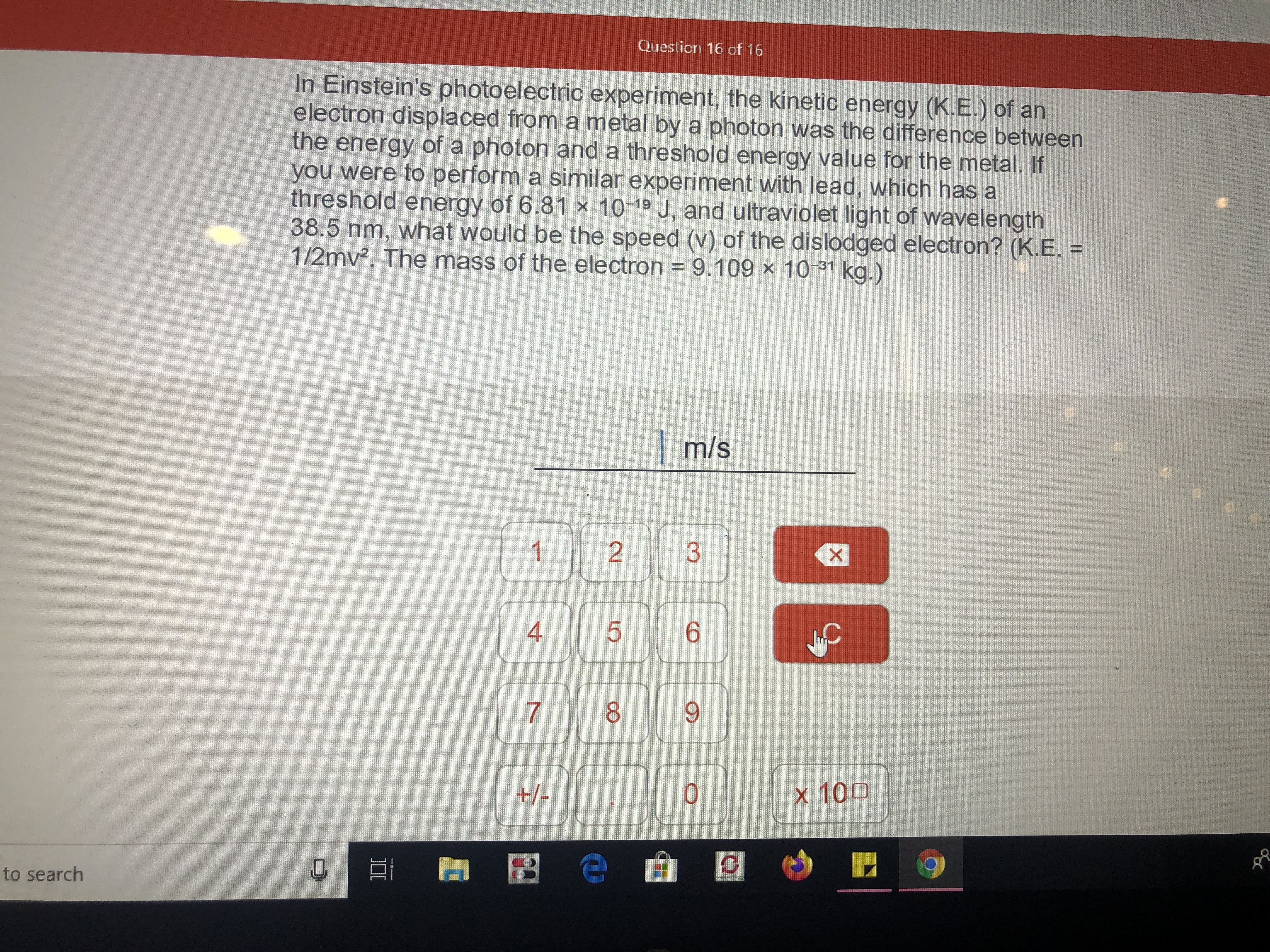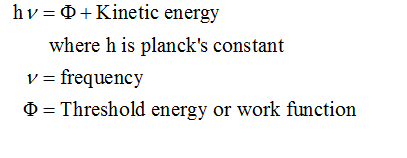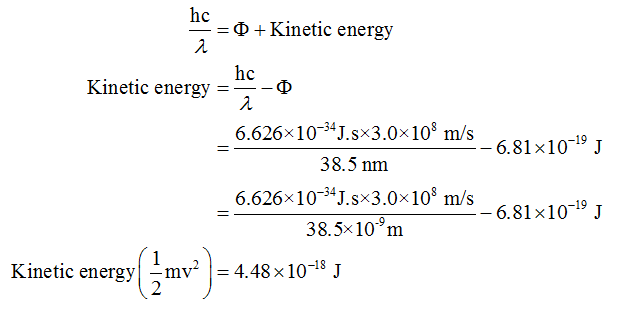# Question 16 of 16In Einstein's photoelectric experiment, the kinetic energy (K.E.) of anelectron displaced from a metal by a photon was the difference betweenthe energy of a photon and a threshold energy value for the metal. Ifyou were to perform a similar experiment with lead, which has athreshold energy of 6.81 × 10 19 J, and ultraviolet light of wavelength38.5 nm, what would be the speed (v) of the dislodged electron? (K.E. =1/2mv². The mass of the electron = 9.109 × 10 1 kg.)m/s4x 100+/-to search2.3.

Question
254 viewshelp_outlineImage TranscriptioncloseQuestion 16 of 16 In Einstein's photoelectric experiment, the kinetic energy (K.E.) of an electron displaced from a metal by a photon was the difference between the energy of a photon and a threshold energy value for the metal. If you were to perform a similar experiment with lead, which has a threshold energy of 6.81 × 10 19 J, and ultraviolet light of wavelength 38.5 nm, what would be the speed (v) of the dislodged electron? (K.E. = 1/2mv². The mass of the electron = 9.109 × 10 1 kg.) m/s 4 x 100 +/- to search 2. 3. fullscreen
check_circle

Step 1

Given information:

Work function of lead = 6.81×10-19 J

Wavelength of light = 38.5 nm

Step 2

When light is radiated on a metal surface then there is an ejection of electrons with the release of some amount of energy. The expression for the photoelectric effect is represented as follows:Step 3

The frequency can be expressed in terms of speed of light (c) and wavelength of light. Therefore, the energy of ejected electrons can be calculated as follows:...

### Want to see the full answer?

See Solution

#### Want to see this answer and more?

Solutions are written by subject experts who are available 24/7. Questions are typically answered within 1 hour.*

See Solution
*Response times may vary by subject and question.
Tagged in